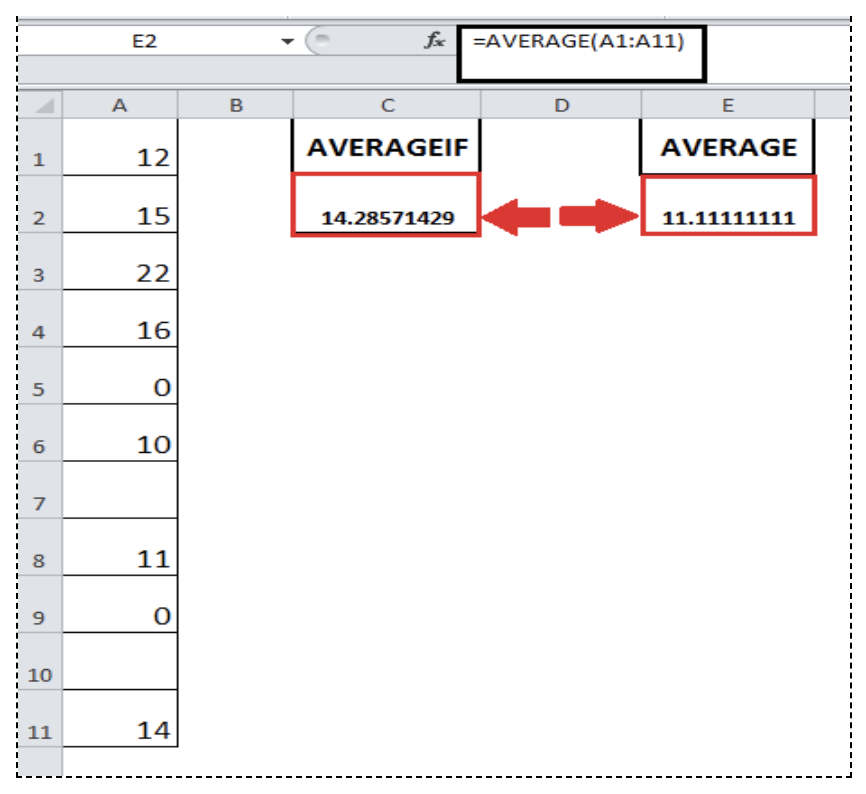Get instant live expert help with Excel or Google Sheets“My Excelchat expert helped me in less than 20 minutes, saving me what would have been 5 hours of work!”

#### Post your problem and you’ll get expert help in seconds.

Your message must be at least 40 characters
Our professional experts are available now. Your privacy is guaranteed.

# How to Calculate an Average Without Zeros or Blank Cells

Excel has special functions to calculate the average of the number in a range of cells and also calculate the average of cells based on specified criteria, like AVERAGE and AVERAGEIF functions. But there are situations where cells in a range are Blank or may contain zeros, so it may affect the result. So you need to take Excel average without zeros and average if not blank. In this case, you need to use AVERAGEIF function to average cells based on criteria.

### The AVERAGEIF function in Excel

AVERAGEIF function averages cells based on supplied criteria in Excel as per its syntax. The syntax of AVERAGEIF function is as follows;

`=AVERAGEIF (range, criteria, [average_range])`

Here,

range – This argument contains the range of cells on which criteria are tested.

criteria – This argument contains the condition to determine which cells to average. It may contain numeric or text values, a logical expression, cell reference or other functions as the condition to meet.

average_range – This argument consists of the range of cells that contain numbers you want to average. It is the optional argument and if you omit this argument in function then function averages cells given in range argument.

### Ignore zeros when finding the average

In this case, as you need to average ignoring zeros in a range argument, so you do not need to supply average_range argument in the AVERAGEIF function here. You need to supply only range argument and criteria argument to find the average.

In the criteria argument, you need to test the criteria “Not Equal to Zero”. This is done by using logical expression Not Equal To (<>) with Zero, enclosed in double quotation marks (“”).

### Ignore Blanks When Finding the Average

Remember that like AVERAGE function, AVERAGEIF function automatically ignores Blank cells and cells containing text values. So you do not need to make any special arrangements to average ignoring blanks and text values in the range. But where cells contain zeros, you need to use AVERAGEIF function based on criteria “Not Equal to Zero.”

In this example you need Excel average ignoring Zeros and Blank cells and how it is different from the simple average of these cells. Suppose you have a range of cells A1:A11 that contains numbers, zeros, and blank cells. By using the AVERAGEIF function with criteria expression Not Equal to Zero (“<>0”) you will average cells ignoring zero and Blank values. The formula, in this case, would be;

`=AVERAGEIF(A1:A11,"<>0") `

This formula eliminates zero values as a result of the criteria expression and Blank cells as default functionality of AVERAGEIF function, so it only counts cells in Excel average without zeros and average if not blank.

As you are aware that AVERAGE function by default ignores Blank cells, but it does not ignore zeros, so that why its result is different from the AVERAGEIF function ignoring zeros and blanks. You can see the difference of results by averaging the same range of values A1:A11 as a result of these two functions, such as;

`=AVERAGE(A1:A11)`

AND

`=AVERAGEIF(A1:A11,"<>0")`

Still need some help with Excel formatting or have other questions about Excel? Connect with a live Excel expert here for some 1 on 1 help. Your first session is always free.Are you still looking for help with the Average function? View our comprehensive round-up of Average function tutorials here.

### Did this post not answer your question? Get a solution from connecting with the expert.Another blog reader asked this question today on Excelchat:
Related blogs
Solution examplesI need help calculating average time elapsed. It is in dd:hh:mm:ss text format and so I can't calculate using =AVERAGEIF
Solved by O. E. in 11 minsI contacted you yesterdaya bout how to use the =AVERAGE function, and for real numbers it works fine, but I tried to get the expert to explain to me how to use the =AVERAGE function when the cells have formulas in them that generate '0' value, so the AVERAGE function would ignore these cells in the averaging method, but got no answer. So, I need to know how use the =AVERAGE function so that when there are formulas in the selected cells to average, that EXCEL would ignore those cells like they have real numbers in them, which seems to work fine. Please advise...
Solved by C. J. in 30 minsI want to calculate an average of some values in a pivot table, however I cannot do that as some rows contain blanks.
Solved by I. C. in 58 minswondering if there is a formula that when the name in one cell changes, the formula in the other cell changes? for example: working on a calculating cost for harvesting grain crops based on acres, bushels harvested, and bushels harvested over x/bushels per acre. in cell B3 i want when cell A1 says "wheat harvest" it will subtract 20 from cell B2, but when cell A1 says "corn harvest" i want to subtract 40 from cell B2 is this possible? thanks
Solved by T. D. in 20 minsHelp, I need a formula to measure if date entered in column e, is between date in column a and date in column b. please
Solved by V. U. in 20 mins## Subscribe to Excelchat.coAnother blog reader asked this question today on Excelchat: